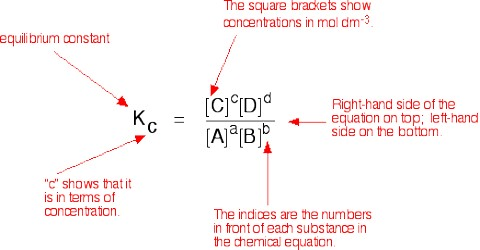# The Equilibrium Law: the Equilibrium Constant

The Equilibrium Law: the Equilibrium Constant

Chemical equilibrium is when the concentrations of the products and the reactants in a reaction are in balance; there is no net exchange as the rate of the forward reaction is equal to the backward reaction. The equilibrium constant of a chemical reaction is the value of the reaction quotient when the reaction has reached equilibrium.

Let us consider a simple reversible reaction of the type

aA + bB ↔ lL + m M … … … (1)

Let [A], [B], [C] and [D] represent the molar concentration of A, B, C and D respectively at any instant. It is possible to show experimentally that at equilibrium,

KC = {[L]le x [M]me} /{ [A]ae x [B]be}

where the subscript e indicates concentrations at the equilibrium position. This is the mathematical expression for the law of chemical equilibrium or simply the equilibrium law. The law states that the equilibrium constant of a reaction at a given temperature equals the product of the equilibrium concentrations of the reaction products divided by the product of the equilibrium concentrations of the reactants, each concentrations being raised to the power equal to the coefficient of the species (molecules of ions) in the balanced equation.The constant KC is called the equilibrium constant and applies to reactions in solution.

The right hand side of the above equation is the equilibrium quotient. Note that it contains only concentration terms. It should not include terms for; (i) any pure solid, (ii) any pure liquid, unless it is a reactant or product of the reaction, or (iii) any solvent.

The equilibrium constant is a true constant for a given reaction. Its value does not depend on the initial concentrations of the reactants or products. The value of the equilibrium constant does, however, vary with temperature.

As the equilibrium constant expression contains concentration terms it will have a unit. The unit will depend on the units of all the concentration terms.